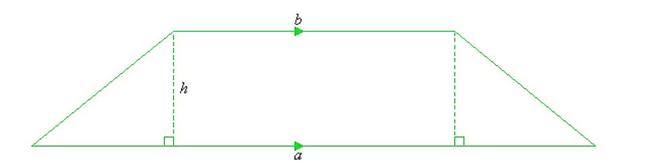Open in App
Not now

# Area of Trapezium – Mensuration | Class 8 Maths

• Last Updated : 11 Dec, 2020

Mensuration is the branch of mathematics that deals with the study of Geometric shapes, their area, volume, and other related parameters. A four-sided closed figure in which one pair of parallel sides opposite to each other and another non-pair of non-parallel sides is called a trapezium.### Properties of Trapezium

• It is a Four-sided Closed Figure with a sum of interior angles 360°.
• One pair of Parallel sides which should be opposite to each other.
• One pair of non-parallel sides.
• Sum of the angles of adjacent sides is 180°.
• Diagonals of a trapezium bisect each other on intersection.

### Basic Terminology for Trapezium

Base of a Trapezium: The pair of parallel sides which are opposite to each other are called base. You can call as b1 and b2 respectively.

Height of a Trapezium: The perpendicular distance between the two parallel lines is called as Height of the trapezium.

### Formula for Area of Trapezium

If the base and height of a trapezium are given, then the area of a Trapezium can be calculated with the help of the formula:Area of Trapezium = 1/2 x (sum of bases) x (Height of trapezium)

### Derivation for Area of a Trapezium

The area of a trapezium is equal to the sum of the areas of the two triangles and the area of the rectangle. Following is the derivation for calculating the area of the trapezium:

Since we know that:

Area of trapezium = Area of triangle 1 + area of rectangle + Area of triangle 2

Let us suppose the base of triangle 1 be B1 and base of triangle 2 is B2 and the height be h for both the triangles. And for rectangle assume its breadth and height be b1 and h.

That means,

A = (B1 x h / 2) + b1h + (B2 x h / 2)

A = (B1 x h + 2b1h + B2 x h) / 2

Simplifying the equation, and rearranging the terms, and factoring result to:

A = h / 2[b1 + (B1 + b1 + B2] ….(i)

If we assume the longer base of the trapezoid be b2, then

b2 = B1 + b1 + B2 …..(ii)

Substituting (ii) in equation (i),

A = h / 2(b1 + b2)

Therefore, the area of a trapezoid with bases b1, b2, and altitude h is;

A = h/2(b1+b2)

which can also be written as below-

Area of Trapezium = 1 / 2 x (sum of bases) x (Height of trapezium)

= 1 / 2 x ( b1 + b2) x h

### Sample Problems based on the Formula

Problem 1: Calculate the area of the trapezium in which the value of bases are 10 and 5 respectively and the height of trapezium is 2 m.

Solution:

Since we know that,

Area of trapezium = 1/2 x (sum of bases) x (Height of trapezium)

= 1/2 x (10 + 5) x 2

= 15 m^2

Problem 2: Given the area of trapezium as 120 m^2 and height of trapezium is 6m and one of base is 4m. Calculate the length of the other base.

Solution:

Area of trapezium = 1/2 x(sum of bases) x ( Height of trapezium)

Let value of other base is b2

Putting all the given values in the above formula, we got

120 = 1/2 x(4+b2) x 6

120 = (4+b2) x3

40 = (4+b2)

b2 = 36 meter

Problem 3: Given the area of trapezium as 220 m^2 and one base is 6m and another base is 4m. Calculate the height of the trapezium.

Solution:

Area of trapezium = 1/2 x  (sum of bases) x ( Height of trapezium)

Let value of height be h.

Putting all the given values in the above formula, we got

220 = 1/2 x (4 + 6) x h

220 = 10 x h

22 = h

h = 22m

My Personal Notes arrow_drop_up
Related Articles